Home » 45 Questions to test a data scientist on basics of Deep Learning (along with solution)

# 45 Questions to test a data scientist on basics of Deep Learning (along with solution)

## Introduction

Back in 2009, deep learning was only an emerging field. Only a few people recognised it as a fruitful area of research. Today, it is being used for developing applications which were considered difficult or impossible to do till some time back.

Speech recognition, image recognition, finding patterns in a dataset, object classification in photographs, character text generation, self-driving cars and many more are just a few examples. Hence it is important to be familiar with deep learning and its concepts.

In this skilltest, we tested our community on basic concepts of Deep Learning. A total of 1070 people participated in this skill test.

If you missed taking the test, here is your opportunity to look at the questions and check your skill level. If you are just getting started with Deep Learning, here is a course to assist you in your journey to Master Deep Learning: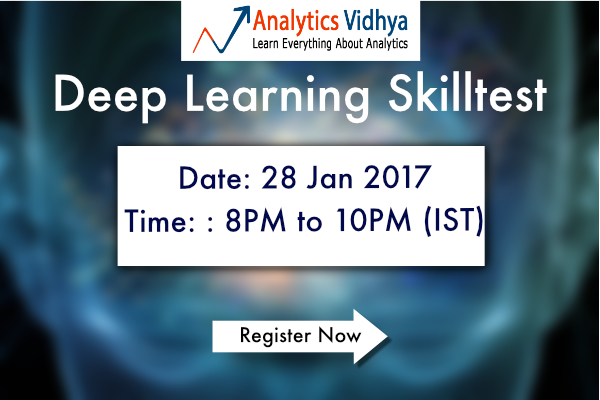## Overall Results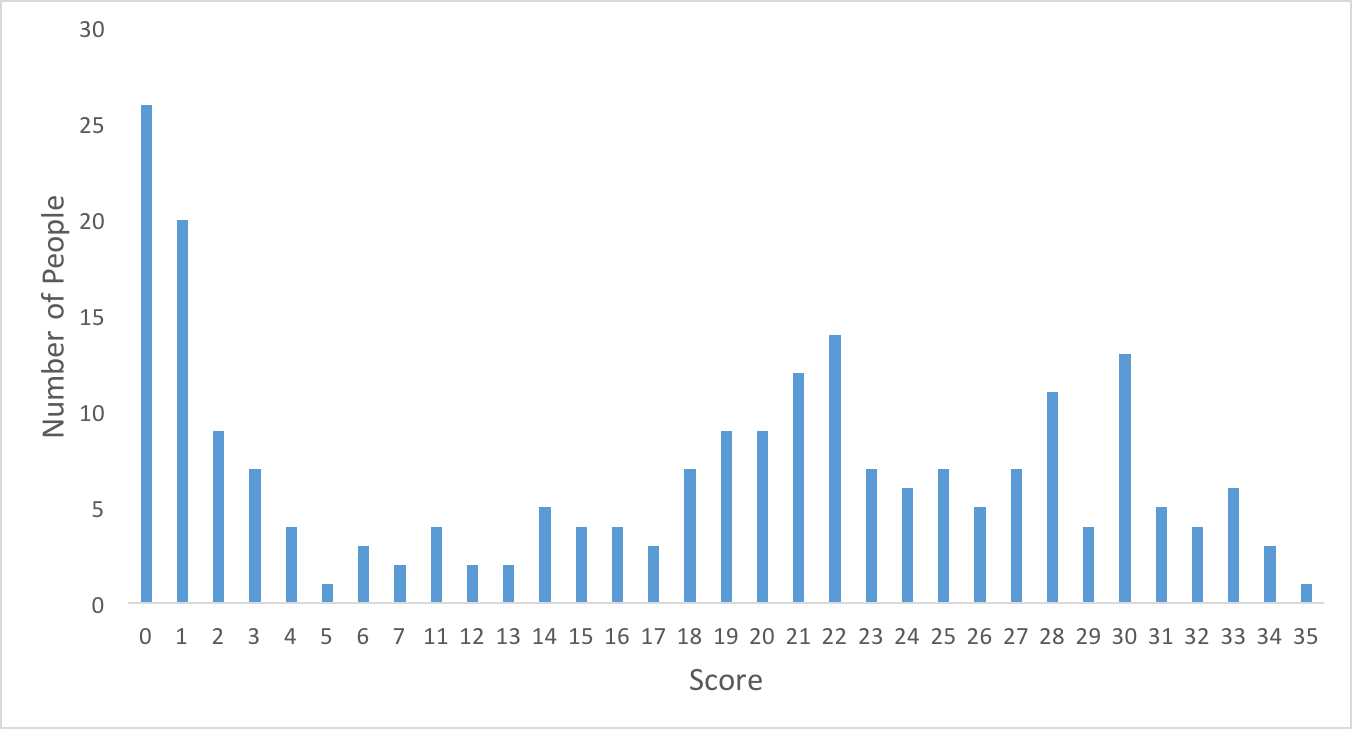You can access your performance here. More than 200 people participated in the skill test and the highest score was 35. Here are a few statistics about the distribution.

Overall distribution

Mean Score: 16.45

Median Score: 20

Mode Score: 0

It seems like a lot of people started the competition very late or didn’t take it beyond a few questions. I am not completely sure why, but may be because the subject is advanced for a lot of audience.

If you have any insight on why this is so, do let us know.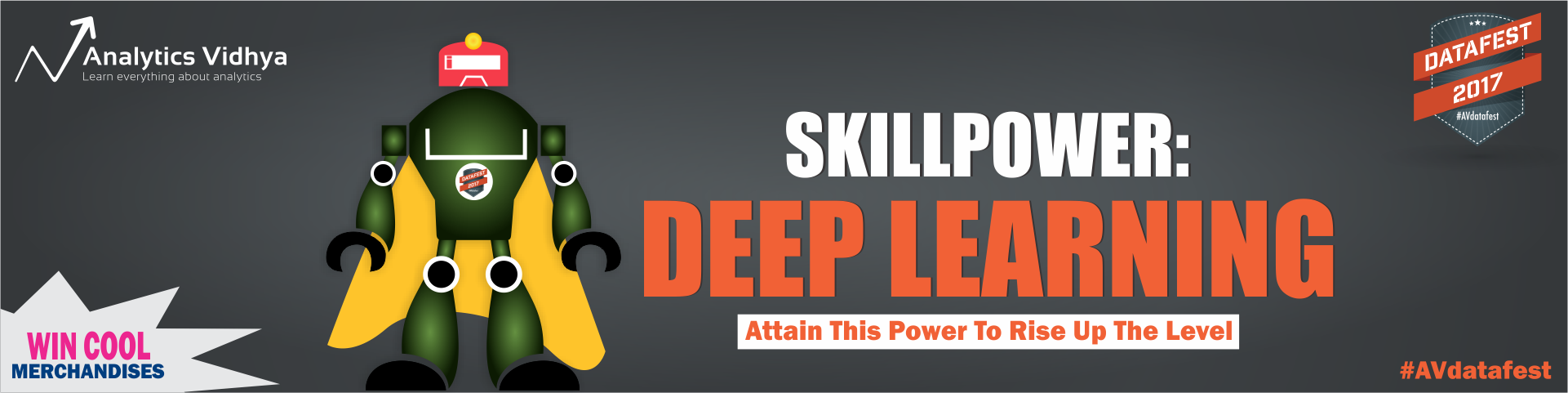Fundamentals of Deep Learning – Starting with Artificial Neural Network

Practical Guide to implementing Neural Networks in Python (using Theano)

A Complete Guide on Getting Started with Deep Learning in Python

Tutorial: Optimizing Neural Networks using Keras (with Image recognition case study)

An Introduction to Implementing Neural Networks using TensorFlow

Q1. A neural network model is said to be inspired from the human brain.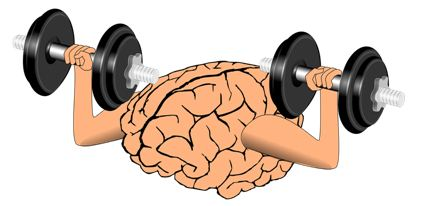The neural network consists of many neurons, each neuron takes an input, processes it and gives an output. Here’s a diagrammatic representation of a real neuron.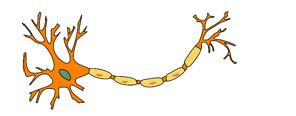Which of the following statement(s) correctly represents a real neuron?

A. A neuron has a single input and a single output only

B. A neuron has multiple inputs but a single output only

C. A neuron has a single input but multiple outputs

D. A neuron has multiple inputs and multiple outputs

E. All of the above statements are valid

Q2. Below is a mathematical representation of a neuron.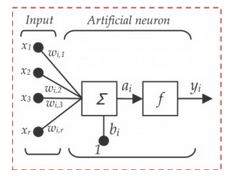The different components of the neuron are denoted as:

• x1, x2,…, xN: These are inputs to the neuron. These can either be the actual observations from input layer or an intermediate value from one of the hidden layers.
• w1, w2,…,wN: The Weight of each input.
• bi: Is termed as Bias units. These are constant values added to the input of the activation function corresponding to each weight. It works similar to an intercept term.
• a:  Is termed as the activation of the neuron which can be represented as
• and y: is the output of the neuron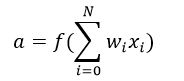Considering the above notations, will a line equation (y = mx + c) fall into the category of a neuron?

A. Yes

B. No

Q3. Let us assume we implement an AND function to a single neuron. Below is a tabular representation of an AND function:

 X1 X2 X1 AND X2 0 0 0 0 1 0 1 0 0 1 1 1

The activation function of our neuron is denoted as: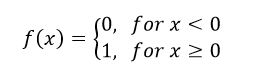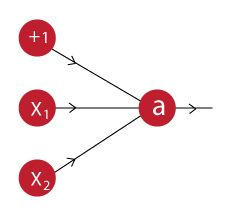What would be the weights and bias?

(Hint: For which values of w1, w2 and b does our neuron implement an AND function?)

A. Bias = -1.5, w1 = 1, w2 = 1

B. Bias = 1.5, w1 = 2, w2 = 2

C. Bias = 1, w1 = 1.5, w2 = 1.5

D. None of these

Q4. A network is created when we multiple neurons stack together. Let us take an example of a neural network simulating an XNOR function.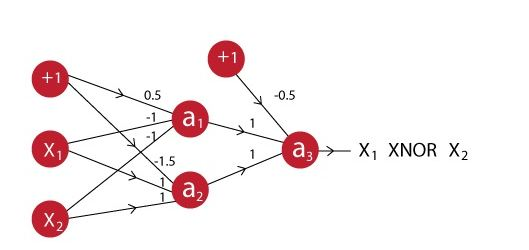You can see that the last neuron takes input from two neurons before it. The activation function for all the neurons is given by:Suppose X1 is 0 and X2 is 1, what will be the output for the above neural network?

A. 0

B. 1

Q5. In a neural network, knowing the weight and bias of each neuron is the most important step. If you can somehow get the correct value of weight and bias for each neuron, you can approximate any function. What would be the best way to approach this?

A. Assign random values and pray to God they are correct

C. Iteratively check that after assigning a value how far you are from the best values, and slightly change the assigned values values to make them better

D. None of these

Q6. What are the steps for using a gradient descent algorithm?

1. Calculate error between the actual value and the predicted value
2. Reiterate until you find the best weights of network
3. Pass an input through the network and get values from output layer
4. Initialize random weight and bias
5. Go to each neurons which contributes to the error and change its respective values to reduce the error

A. 1, 2, 3, 4, 5

B. 5, 4, 3, 2, 1

C. 3, 2, 1, 5, 4

D. 4, 3, 1, 5, 2

Q7. Suppose you have inputs as x, y, and z with values -2, 5, and -4 respectively. You have a neuron ‘q’ and neuron ‘f’ with functions:

q = x + y

f = q * z

Graphical representation of the functions is as follows: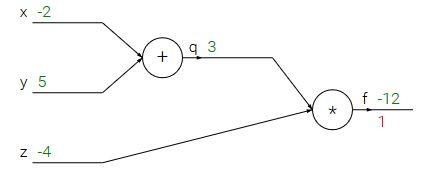What is the gradient of F with respect to x, y, and z?

(HINT: To calculate gradient, you must find (df/dx), (df/dy) and (df/dz))

A. (-3,4,4)

B. (4,4,3)

C. (-4,-4,3)

D. (3,-4,-4)

Q8. Now let’s revise the previous slides. We have learned that:

• A neural network is a (crude) mathematical representation of a brain, which consists of smaller components called neurons.
• Each neuron has an input, a processing function, and an output.
• These neurons are stacked together to form a network, which can be used to approximate any function.

Given above is a description of a neural network. When does a neural network model become a deep learning model?

A. When you add more hidden layers and increase depth of neural network

B. When there is higher dimensionality of data

C. When the problem is an image recognition problem

D. None of these

Q9. A neural network can be considered as multiple simple equations stacked together. Suppose we want to replicate the function for the below mentioned decision boundary.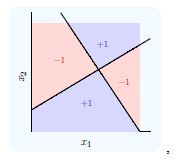Using two simple inputs h1 and h2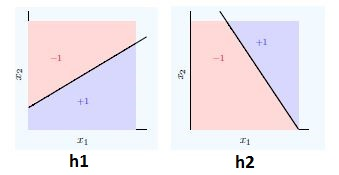What will be the final equation?

A. (h1 AND NOT h2) OR (NOT h1 AND h2)

B. (h1 OR NOT h2) AND (NOT h1 OR h2)

C. (h1 AND h2) OR (h1 OR h2)

D. None of these

Q10. “Convolutional Neural Networks can perform various types of transformation (rotations or scaling) in an input”. Is the statement correct True or False?

A. True

B. False

Q11. Which of the following techniques perform similar operations as dropout in a neural network?

A. Bagging

B. Boosting

C. Stacking

D. None of these

Q 12. Which of the following gives non-linearity to a neural network?

B. Rectified Linear Unit

C. Convolution function

D. None of the above

Q13. In training a neural network, you notice that the loss does not decrease in the few starting epochs.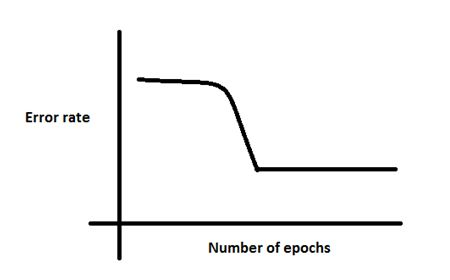The reasons for this could be:

1. The learning is rate is low
2. Regularization parameter is high
3. Stuck at local minima

What according to you are the probable reasons?

A. 1 and 2

B. 2 and 3

C. 1 and 3

D. Any of these

Q14. Which of the following is true about model capacity (where model capacity means the ability of neural network to approximate complex functions) ?

A. As number of hidden layers increase, model capacity increases

B. As dropout ratio increases, model capacity increases

C. As learning rate increases, model capacity increases

D. None of these

Q15. If you increase the number of hidden layers in a Multi Layer Perceptron, the classification error of test data always decreases. True or False?

A. True

B. False

Q16. You are building a neural network where it gets input from the previous layer as well as from itself.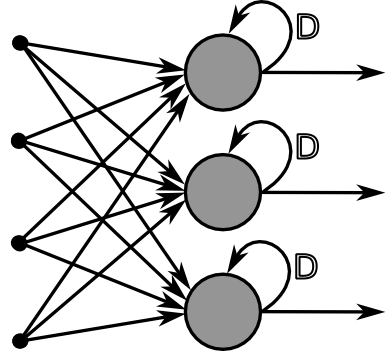Which of the following architecture has feedback connections?

A. Recurrent Neural network

B. Convolutional Neural Network

C. Restricted Boltzmann Machine

D. None of these

Q17. What is the sequence of the following tasks in a perceptron?

1. Initialize weights of perceptron randomly
2. Go to the next batch of dataset
3. If the prediction does not match the output, change the weights
4. For a sample input, compute an output

A. 1, 2, 3, 4

B. 4, 3, 2, 1

C. 3, 1, 2, 4

D. 1, 4, 3, 2

Q18. Suppose that you have to minimize the cost function by changing the parameters. Which of the following technique could be used for this?

A. Exhaustive Search

B. Random Search

C. Bayesian Optimization

D. Any of these

Q19. First Order Gradient descent would not work correctly (i.e. may get stuck) in which of the following graphs?

A.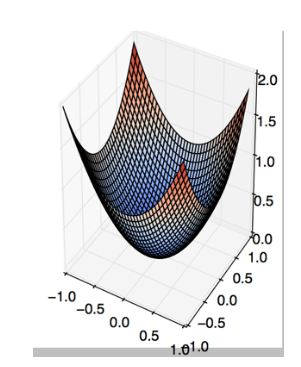B.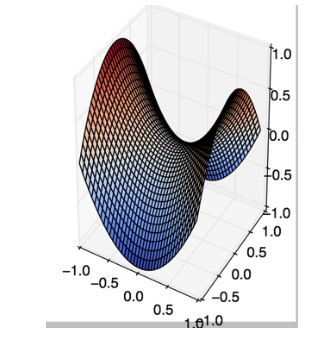C.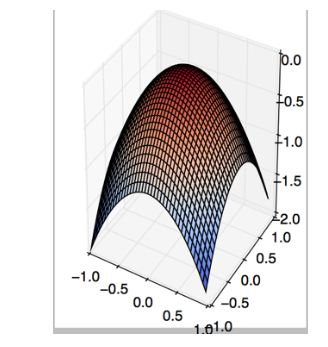D. None of these

Q20. The below graph shows the accuracy of a trained 3-layer convolutional neural network vs the number of parameters (i.e. number of feature kernels).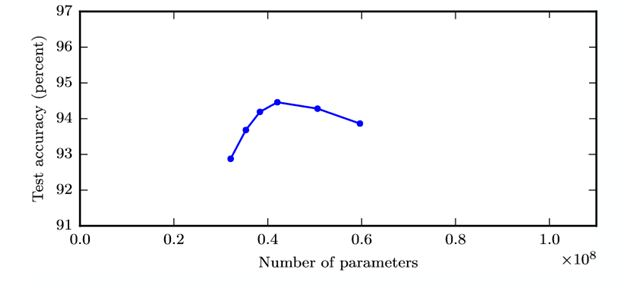The trend suggests that as you increase the width of a neural network, the accuracy increases till a certain threshold value, and then starts decreasing.

What could be the possible reason for this decrease?

A. Even if number of kernels increase, only few of them are used for prediction

B. As the number of kernels increase, the predictive power of neural network decrease

C. As the number of kernels increase, they start to correlate with each other which in turn helps overfitting

D. None of these

Q21. Suppose we have one hidden layer neural network as shown above. The hidden layer in this network works as a dimensionality reductor. Now instead of using this hidden layer, we replace it with a dimensionality reduction technique such as PCA.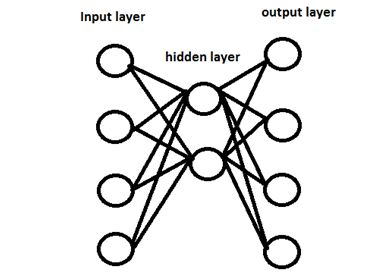Would the network that uses a dimensionality reduction technique always give same output as network with hidden layer?

A. Yes

B. No

Q22. Can a neural network model the function (y=1/x)?

A. Yes

B. No

Q23. In which neural net architecture, does weight sharing occur?

A. Convolutional neural Network

B. Recurrent Neural Network

C. Fully Connected Neural Network

D. Both A and B

Q24. Batch Normalization is helpful because

A. It normalizes (changes) all the input before sending it to the next layer

B. It returns back the normalized mean and standard deviation of weights

C. It is a very efficient backpropagation technique

D. None of these

Q25. Instead of trying to achieve absolute zero error, we set a metric called bayes error which is the error we hope to achieve. What could be the reason for using bayes error?

A. Input variables may not contain complete information about the output variable

B. System (that creates input-output mapping) may be stochastic

C. Limited training data

D. All the above

Q26. The number of neurons in the output layer should match the number of classes (Where the number of classes is greater than 2) in a supervised learning task. True or False?

A. True

B. False

Q27. In a neural network, which of the following techniques is used to deal with overfitting?

A. Dropout

B. Regularization

C. Batch Normalization

D. All of these

Q28. Y = ax^2 + bx + c (polynomial equation of degree 2)

Can this equation be represented by a neural network of single hidden layer with linear threshold?

A. Yes

B. No

Q29. What is a dead unit in a neural network?

A. A unit which doesn’t update during training by any of its neighbour

B. A unit which does not respond completely to any of the training patterns

C. The unit which produces the biggest sum-squared error

D. None of these

Q30. Which of the following statement is the best description of early stopping?

A. Train the network until a local minimum in the error function is reached

B. Simulate the network on a test dataset after every epoch of training. Stop training when the generalization error starts to increase

C. Add a momentum term to the weight update in the Generalized Delta Rule, so that training converges more quickly

D. A faster version of backpropagation, such as the `Quickprop’ algorithm

Q31. What if we use a learning rate that’s too large?

A. Network will converge

B. Network will not converge

C. Can’t Say

Q32. The network shown in Figure 1 is trained to recognize the characters H and T as shown below: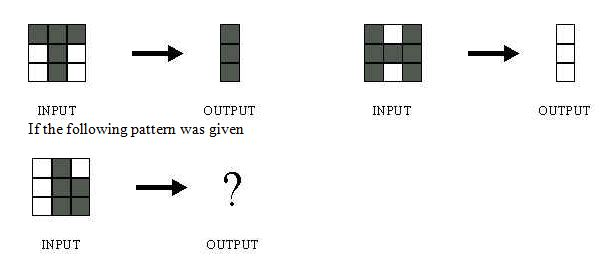What would be the output of the network?

1.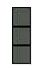2.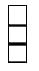3.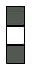4. Could be A or B depending on the weights of neural network

Q33. Suppose a convolutional neural network is trained on ImageNet dataset (Object recognition dataset). This trained model is then given a completely white image as an input.The output probabilities for this input would be equal for all classes. True or False?

A. True

B. False

Q34. When pooling layer is added in a convolutional neural network, translation in-variance is preserved. True or False?

A. True

B. False

Q35. Which gradient technique is more advantageous when the data is too big to handle in RAM simultaneously?

Q36. The graph represents gradient flow of a four-hidden layer neural network which is trained using sigmoid activation function per epoch of training. The neural network suffers with the vanishing gradient problem.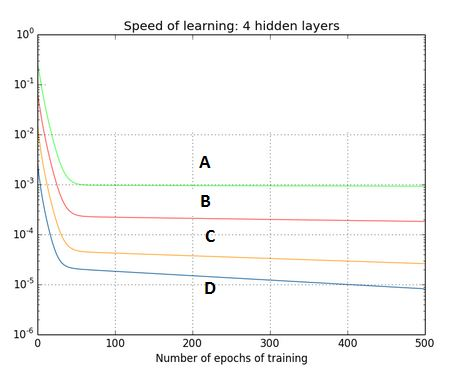Which of the following statements is true?

A. Hidden layer 1 corresponds to D, Hidden layer 2 corresponds to C, Hidden layer 3 corresponds to B and Hidden layer 4 corresponds to A

B. Hidden layer 1 corresponds to A, Hidden layer 2 corresponds to B, Hidden layer 3 corresponds to C and Hidden layer 4 corresponds to D

Q37. For a classification task, instead of random weight initializations in a neural network, we set all the weights to zero. Which of the following statements is true?

A. There will not be any problem and the neural network will train properly

B. The neural network will train but all the neurons will end up recognizing the same thing

C. The neural network will not train as there is no net gradient change

D. None of these

Q38. There is a plateau at the start. This is happening because the neural network gets stuck at local minima before going on to global minima.To avoid this, which of the following strategy should work?

A. Increase the number of parameters, as the network would not get stuck at local minima

B. Decrease the learning rate by 10 times at the start and then use momentum

C. Jitter the learning rate, i.e. change the learning rate for a few epochs

D. None of these

Q39. For an image recognition problem (recognizing a cat in a photo), which architecture of neural network would be better suited to solve the problem?

A. Multi Layer Perceptron

B. Convolutional Neural Network

C. Recurrent Neural network

D. Perceptron

Q40. Suppose while training, you encounter this issue. The error suddenly increases after a couple of iterations.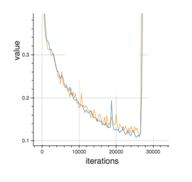You determine that there must a problem with the data. You plot the data and find the insight that, original data is somewhat skewed and that may be causing the problem.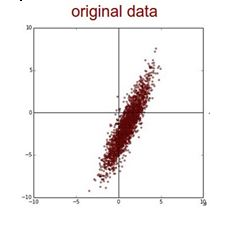What will you do to deal with this challenge?

A. Normalize

B. Apply PCA and then Normalize

C. Take Log Transform of the data

D. None of these

Q41. Which of the following is a decision boundary of Neural Network?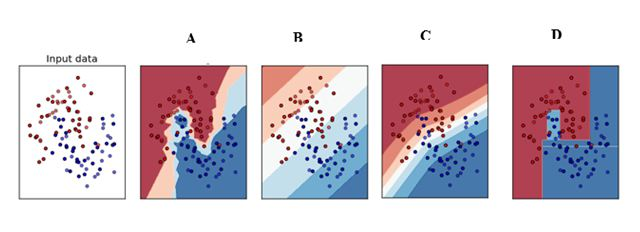A) B

B) A

C) D

D) C

E) All of these

Q42. In the graph below, we observe that the error has many “ups and downs”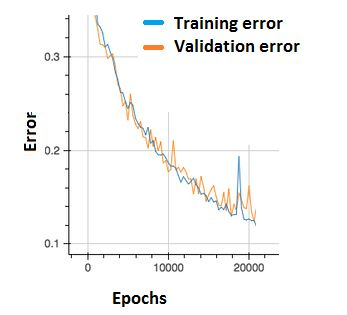Should we be worried?

A. Yes, because this means there is a problem with the learning rate of neural network.

B. No, as long as there is a cumulative decrease in both training and validation error, we don’t need to worry.

Q43. What are the factors to select the depth of neural network?

1. Type of neural network (eg. MLP, CNN etc)
2. Input data
3. Computation power, i.e. Hardware capabilities and software capabilities
4. Learning Rate
5. The output function to map

A. 1, 2, 4, 5

B. 2, 3, 4, 5

C. 1, 3, 4, 5

D. All of these

Q44. Consider the scenario. The problem you are trying to solve has a small amount of data. Fortunately, you have a pre-trained neural network that was trained on a similar problem. Which of the following methodologies would you choose to make use of this pre-trained network?

A. Re-train the model for the new dataset

B. Assess on every layer how the model performs and only select a few of them

C. Fine tune the last couple of layers only

D. Freeze all the layers except the last, re-train the last layer

Q45. Increase in size of a convolutional kernel would necessarily increase the performance of a convolutional network.

A. True

B. False

## End Notes

I hope you enjoyed taking the test and you found the solutions helpful. The test focused on conceptual knowledge of Deep Learning.

We tried to clear all your doubts through this article but if we have missed out on something then let me know in comments below. If you have any suggestions or improvements you think we should make in the next skilltest, let us know by dropping your feedback in the comments section.

### Learn, compete, hack and get hired!

You can also read this article on our Mobile APP•Mohan Chakradhar Vedurupaka says:

For Q31. “What if we use a learning rate that’s too large?”, only three options (A,B,C) are available. But the answer is mentioned as E. I think options D, E are missing.

•Faizan Shaikh says:

Thanks for pointing it out; Corrected it

•Dai Zhongxiang says:

Thanks for the post. Can you provide more explanation on Q14? why would the model capacity decrease as the number of hidden layers increase? Intuitively, shouldn’t it be the other way around?

Thanks.

•Faizan Shaikh says:

Hey. First of all, congrats on being on the top of leaderboard!

Thanks for pointing out the typo; You are right, model capacity increases on increasing hidden layers. A prime example of this can be seen in visualizing Convolutional Neural nets in image recognition problems, where the starting layers tend to recognize basics shapes like edges and the last layers tend to recognize individual faces

•Dai Zhongxiang says:

I see. Thanks for the clarification 🙂

•Sukuya says:

During the quiz also there was this typo and that is why I marked D which must have been graded wrong then 🙁

•Faizan Shaikh says:

Yes. But no need to worry. It was a practice test right? I too got to learn from it 🙂

•Sukuya says:

Sure. 🙂

•Sukuya says:

Regarding Q1, how can a real neuron have multiple outputs ? When the signal passes through axon, effectively it’s the same signal. If you branch it further, all values will be the same. So, is it appropriate to say that real neurons can have multiple outputs. ?

•Faizan Shaikh says:

This was actually a new information to me too that a real neuron can have multiple outputs. Here’s an excerpt of what researchers found:

“Model organisms usually possess a small nervous system but nevertheless execute a large array of complex behaviors, suggesting that some neurons are likely multifunctional and may encode multiple behavioral outputs. Here, we show that the C. elegans interneuron AIY regulates two distinct behavioral outputs: locomotion speed and direction-switch by recruiting two different circuits.” Source: (http://www.cell.com/cell/abstract/S0092-8674(14)01244-6)

Intuitively speaking, a real neuron is much more complex than a artificial neuron. It implements a “spiking mechanism”, which can encode a lot more complex functions and even multiple functions.

•Sukuya says:

That’s surely a new piece of information. I have not gone through the entire paper but just at the abstract. Multiple functionality is something different than multiple output. At any given time, can we say that a neuron is giving two different outputs ? What I believe is that the paper is talking about how the activation function of a neuron can drastically differ so as to produce different behavior, but not simultaneously. May be, I’ll read more. 🙂

•Faizan Shaikh says:

You are right on the fact that the paper talks about multi-output as a non-simultaneous activity. But can’t it still be considered as a new output, which is inherently different from the previous one?

If you consider simultaneous multiple output as a different output; consider this. In a real neuron, there can be more than one synapse. And each synapse can be affected by many factors; such as refactory period of the synapse, transfer of neurotransmitters between the connections of synapse and the next axon, nature of neuron (inhibitory or excitatory), can depend on the frequency and amplitude of the “spikes”, etc. So the output of a real neuron can be multiple and stochastic. The model of an artificial neuron is indeed a very simplistic view of the real neuron. Here’s a discussion on the topic that might interest you (https://www.reddit.com/r/askscience/comments/2yl5jh/how_does_one_neuron_with_multiple_synapses_decide/)

How does that sound?

•stepherd says:

I simply want to tell you that I’m all new to blogs and truly liked you’re blog site. Very likely I’m likely to bookmark your site .You surely come with remarkable articles. Cheers for sharing your website page.

•Faizan says:

Thanks stepherd!

•Shuaib Ahmed says:

Q26. The number of neurons in the output layer should match the number of classes (Where the number of classes is greater than 2) in a supervised learning task. True or False?
Solution: (A)
The number of output neurons should be exactly equal to number of output classes.

It depends on output encoding. If it is one-hot encoding, then its true. You can have two output for four class, and take the binary values as four classes(00,01,10,11). You can have just one output that takes range in 0-1 scale as four classes(0.00-0.25,0-0.50,0.50-0.75,0.75-1.00) .

•Faizan Shaikh says:

Your explanation seems correct. I should update the article.

Thanks for clarification!